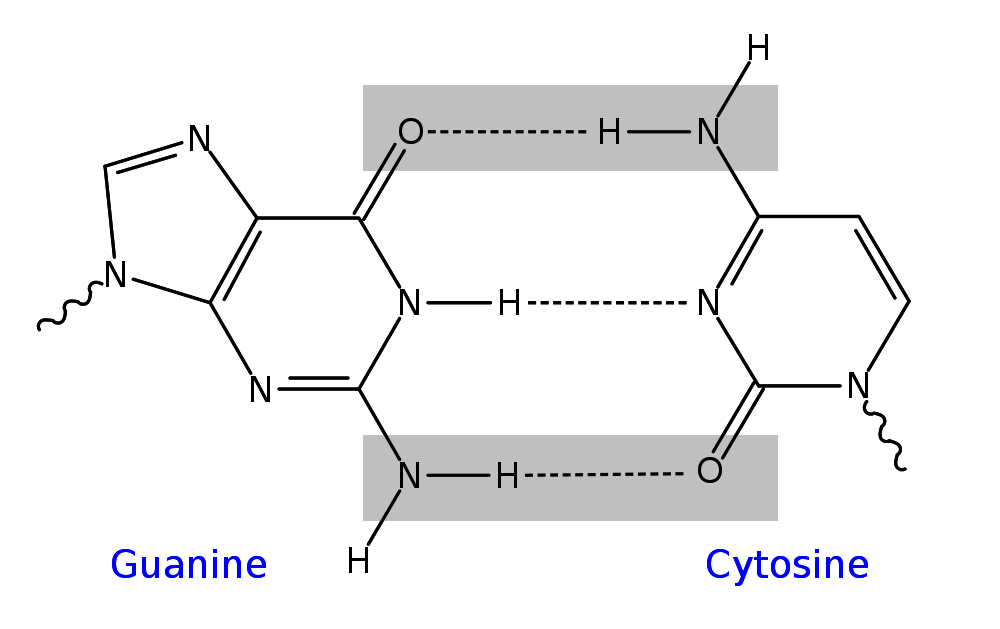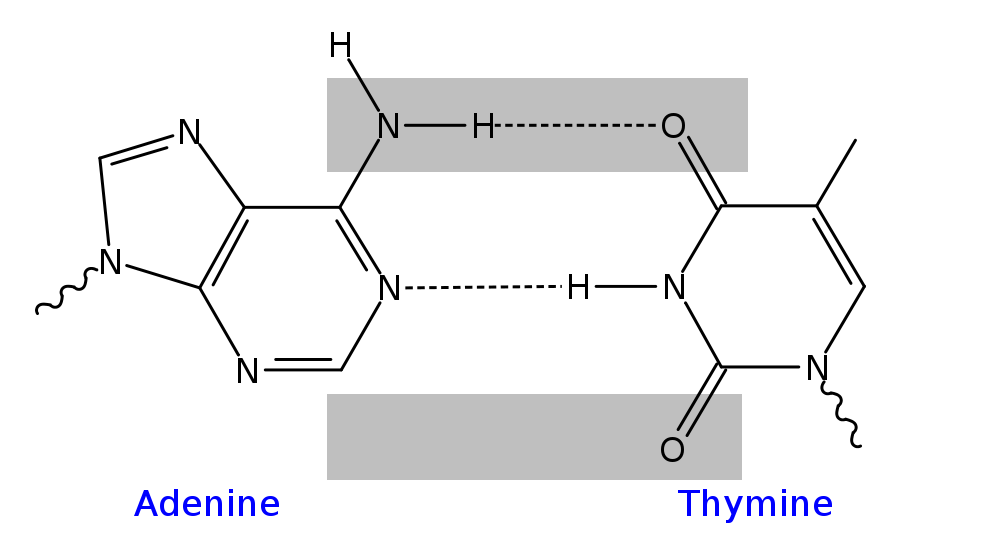# Going Binary

This section demonstrates how the current view our our genetic code can effortlessly be switched to binary.

The current view suggests that there are four different bases on our DNA, Adenine (A), Thymine (T), Guanine (G) and Cytocine (C). Our genetic information is written on our DNA molecule as a sequence of these four letters, in the same way a page of a book is written in a sequence of letters of the alphabet. The fact that we are using four letters is just a convention. We chose to describe the genetic code with these four letters because this was more meaningful to us: each letter gave away additional information about the chemistry of the actual molecule involved. We could be using four numbers, for example instead of A we could use 0, instead of T we could use 1, instead of G we could use 2, and instead of C we could use 3. We could be using four different colours or four different shapes/symbols instead.

The current scientific view is that our DNA is written in a digital and quaternary format.

What if we were to change our view to a digital and binary format, using the following conversion:

 A becomes 0 0 C becomes 0 1 G becomes 1 0 T becomes 1 1

This is one more convention, with an interesting result. We just decided to change the way we are representing the four letters and in the process we converted the 4-letter code into a binary code.

Here is an example: with this new convention, the sequence of the 5 bases ACGTA can be written as 0001101100.

Can this be more than just a convention? Is it possible that nature actually has two binary properties per base? The answer is yes. The presence (1) or absence (0) of a carbon-oxygen bond in two distinct positions on each base explain the above table (with the N-H-N bond providing the read direction). In other words, if we just look at the molecules we see a quaternary code. If we look at the chemical bonds involved, we see a binary code emerging.As you can see from the images above, Adenine has no C=0 bonds present in the two highlighted positions which in binary is indicated as 0 0. Thymine has two C=O bonds, which in binary is written as 1 1. Cytocine and Guanine have one each, at opposing positions, hence in binary these two are shown as 0 1 and 1 0 respectively.

This is just one way of showing how the current quaternary view can be transformed to a binary view, simply by looking at the chemical bonds involved.